# Structure Of Atom- Download Science Study Notes for CTET 2020 FREE PDFIn many teaching exams including CTET 2020, UPTET 2020 STET 2020 etc. Science may be an interesting subject having and 30 questions of Science content and 30 questions of Science Pedagogy in CTET and other State TET Exams. Science comprising of various branches of studies like chemistry, physical science, and life science.

## STRUCTURE OF ATOM

Atoms:
Atoms are the building blocks of matter. It is the smallest unit of matter that is composed of three sub-atomic particles: the proton, the neutron, and the electron.
Earlier Dalton postulated that atom is indivisible i.e. cannot be further divided which proved to be wrong by discovery of sub – atomic particles.

Charged Particles in Matter:
Whenever we rub two objects together, they become electrically charged. This is because atoms contain charged particles in them. Therefore, atoms can be divided further into particles i.e proton, electron and neutron.
• Protons were discovered by Ernest Rutherford, in his famous gold foil experiment.
• Electrons were discovered by J.J. Thomson, in his cathode ray tube experiment.
• Neutrons were discovered by James Chadwick.

Types of Sub – atomic Particles: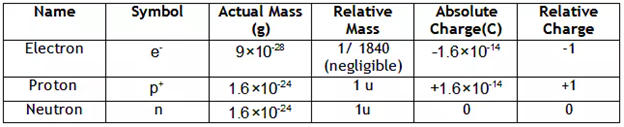Cathode ray experiment:
• J. J. Thomson discovered the existence of electrons.
• He did this using a cathode ray tube, which is a vacuum-sealed tube with a cathode and anode on one end that created a beam of electrons travelling towards the other end of the tube.
• The air inside the chamber is subjected to high voltage and electricity flows through the air from the negative electrode to the positive electrode.
• The characteristics of cathode rays (electrons) do not depend upon the material of electrodes and the nature of the gas present in the cathode ray tube.
• The collection of negatively charged particles emitted from cathode of discharge tube is called cathode rays.

#### How To Score 25+ in Science For CTET Exam

Proton (p+):
• In 1886, Goldstein observed in the same experiment, with different situations that the anode emitted positive particles which were called
• Canal rays: The positively charged radiations produced in the discharge tube from anode are called Canal rays.

Neutron (n):
• These neutrally charged particles were discovered by James Chadwick in 1932. Neutrons are present in atoms of all elements except Hydrogen.

Thomson’s Model of an Atom:
According to J.J. Thomson, the structure of an atom can be compared to Christmas pudding where electrons are present inside a positive sphere.
• An atom is composed of a positively charged sphere in which electrons are embedded.
• Atom is neutral as the positive and negative charged are equal in proportion.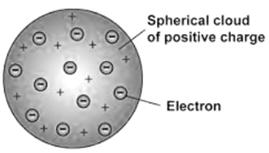Rutherford’s Model of an Atom:
• In this experiment, fast-moving alpha (α)-particles were made to fall on a thin gold foil. His observations were:
i. A major fraction of the α-particles bombarded towards the gold sheet passed through it without any deflection, and hence most of the space in an atom is empty.
ii. Some of the α-particles were deflected by the gold sheet by very small angles, and hence the positive charge in an atom is not uniformly distributed.
iii. The positive charge in an atom is concentrated in a very small volume.
iv. Very few of the α-particles were deflected back, that is only a few α-particles had nearly 180° angle of deflection. So the volume occupied by the positively charged particles in an atom is very small as compared to the total volume of an atom.

• Rutherford concluded the model of the atom from the α-particle scattering experiment as:
(i) There is a positively charged centre in an atom called the nucleus. Nearly all the mass of an atom resides in the nucleus.
(ii) The electrons revolve around the nucleus in well-defined orbits.
(iii) The size of the nucleus is very small as compared to the size of the atom.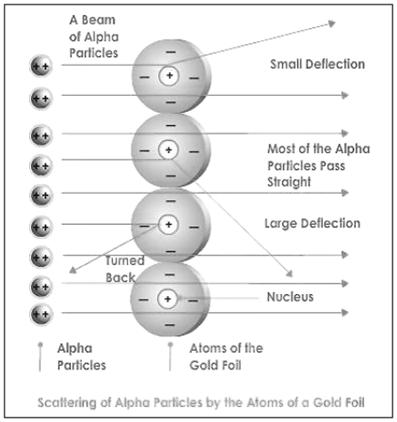OBSERVATION INFERENCE Alpha particles which had high speed moved straight through the gold foil. Atom contains a lot of empty space. Some particles got diverted a by slide angles. Positive charges in the atom are not occupying much of its space. Only one out of 12000 particles bounced back. The positive charges are concentrated over a particular area of the atom.

#### Check Complete Science Study Now HERE

Thus, Rutherford gave the nuclear model of an atom based on his experiment which suggests that –
• Atoms contain a lot of unoccupied space.
• There is a heavily positively charged substance present in the center of the atom which is called the nucleus.
• The nucleus contains an equal amount of positive and negative charge.

The Nucleus of an Atom:
• The nucleus is located at the center of the atom.
• All the mass of the atom is because of the nucleus.
• The electrons revolve around the nucleus in circular parts which are called orbits.
• If we compare the size of the atom and nucleus, the nucleus is much smaller than the atom.

Drawbacks of Rutherford’s model of the atom:
When an electron undergoes acceleration, it radiates energy. Thus revolving electron would lose energy and finally fall into the nucleus.

Bohr’s Model of an Atom:
Bohr suggested that –
• Electrons spin around the nucleus in an individualized separate path or unattached orbit.
• The electrons do not emit any energy while moving Indies special orbits.
• These orbits are also called as Energy Levels.
• They are represented using letters or numbers as shown in the figure below :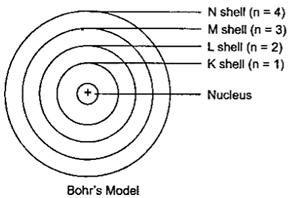The distribution of electrons in different shells or orbits:
• If Orbit number = n
• Then number of electrons present in an Orbit = 2n2
• So, for n =1
• Maximum electrons present in shell – K = 2 * (1)2 = 2
• The outermost shell can contain at most 8 electrons.
• The shells in an atom are filled in sequence.
• Thus, until the inner shells of an atom are filled completely the outer shells cannot contain any electrons.

Valency:
Valence Electrons: Electrons existing in the outermost orbit of an atom are called Valence Electrons.
• The atoms which have completely filled the outermost shell are not very active chemically.
• The valency of an atom or the combining capacity of an atom is given by the number of elements present in the outermost shell.
• For Example, Helium contains two electrons in its outermost shell which means its valency is two. In other words, it can share two electrons to form a chemical bond with another element.

### GET FREE Study Material For CTET 2020 Exam

Atomic Number of an Element:
• The number of protons present in the nucleus of an atom is called atomic number. It is denoted by Z.

Atomic Number (Z) = Number of protons in an atom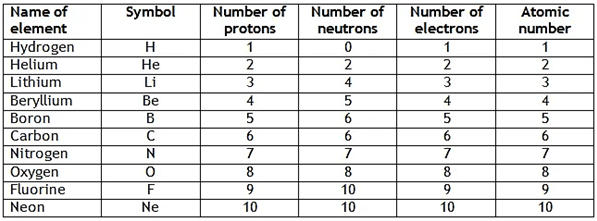Mass Number of an Element:
• Mass number is equal to the number of nucleons present inside the nucleus of an atom. It means it is the sum of number of protons and neutrons present in the nucleus of an atom. It is denoted by letter A.

Mass Number = Number of protons + Number of neutrons
• For Example,
Carbon : Mass number = 12 (no. of p= 6, no. of  n = 6 )
Nitrogen : Mass number = 14 (no. of p= 7, no. of  n = 7 )
Fluorine : Mass number = 19 (no. of p= 9, no. of  n = 10 )

#### Check Complete EVS Study Now: HERE

Isotopes:
• Atoms of the same element with same atomic number but a different mass number are called isotopes.
• Chemical properties → same but Physical properties → different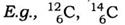(a) Applications of Isotopes:
• An isotope of Uranium used as fuel.
• An isotope of Cobalt is used in the treatment of cancer
• An isotope of Iodine is used in the treatment of goiter.

Isobars:
Atoms of different elements with same mass number but different atomic numbers are called isobars.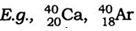•अलंकार - परिभाष�...
•विशेषण- परिभाष�...
•लेखन कौशल की पर�...
•Complete CTET Child Development and Peda...
•New Education Policy(NEP) PDF - नई �...
•पठन कौशल- परिभा�...